## Python groupby 分组 再求平均值 求和 agg聚合 transform不改变形状应用函数_python groupby transform_正在学习中的李斌的博客-程序员秘密

``````一、 groupby 依据某列分组； groupby 依据多列分组；

``````

# 一、分组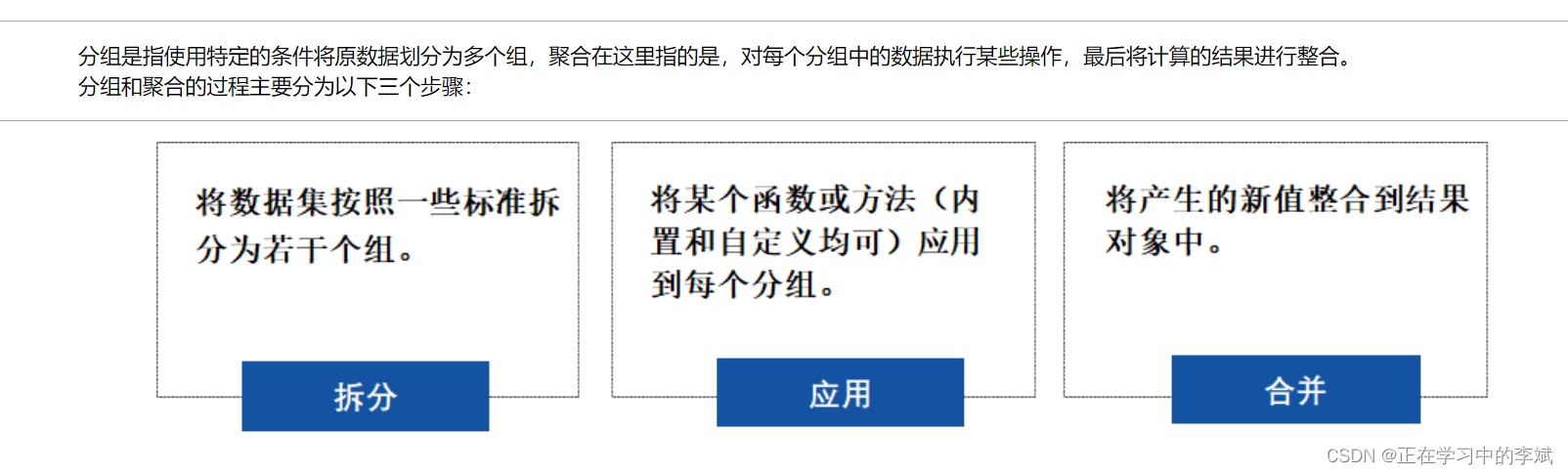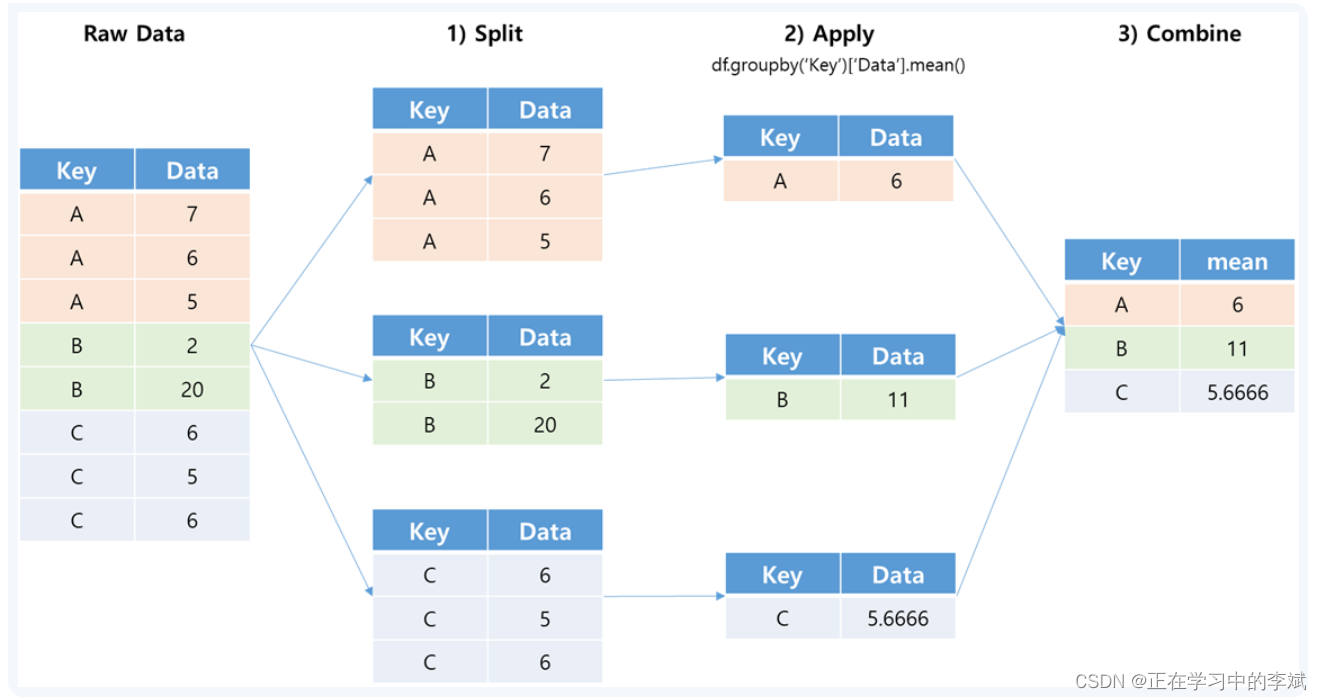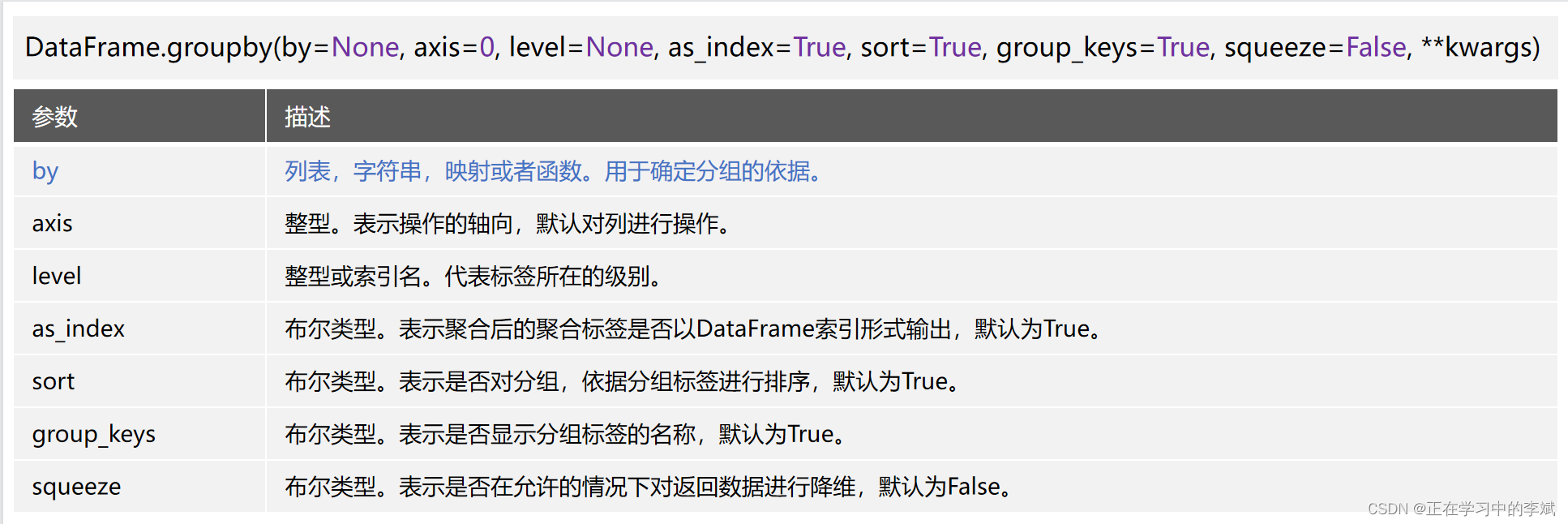``````import pandas as pd
import glob
# 获取该目录下的所有文件
files = glob.glob('../../data/03表格数据处理Pandas/C3.7 数据的分组与聚合/*')
# 利用 concat 将所有数据拼接成一个大的 df
df = pd.concat([pd.read_csv(f) for f in files])
# 删除列（值全为空）；删除行（存在任意空值）
df = df.dropna(axis='columns', how='all').dropna(axis='index', how='any')
# 对 date 这一列进行格式转换
df['date'] = df.apply({
'date': lambda x: pd.to_datetime(x, format='%Y%m%d')})
# 获取到 月 和 天
df['month'] = df['date'].dt.month
df['day'] = df['date'].dt.day
# 删除 date 列
df = df.drop(columns='date')

# 简单分组
# 分组后的数据想要查看，必须循环打印
group = df.groupby('type')
for i in group:
print(i)

# 获取某列元素等于特定值的数据
df[df['type']=='AQI']

# 多重分组
# 分组后的数据想要查看，必须循环打印
group = df.groupby(['type', 'month'])
for i in group:
print(i)

# 获取同时满足多个列条件的数据
df[(df['type']=='AQI') & (df['month']==1)]
``````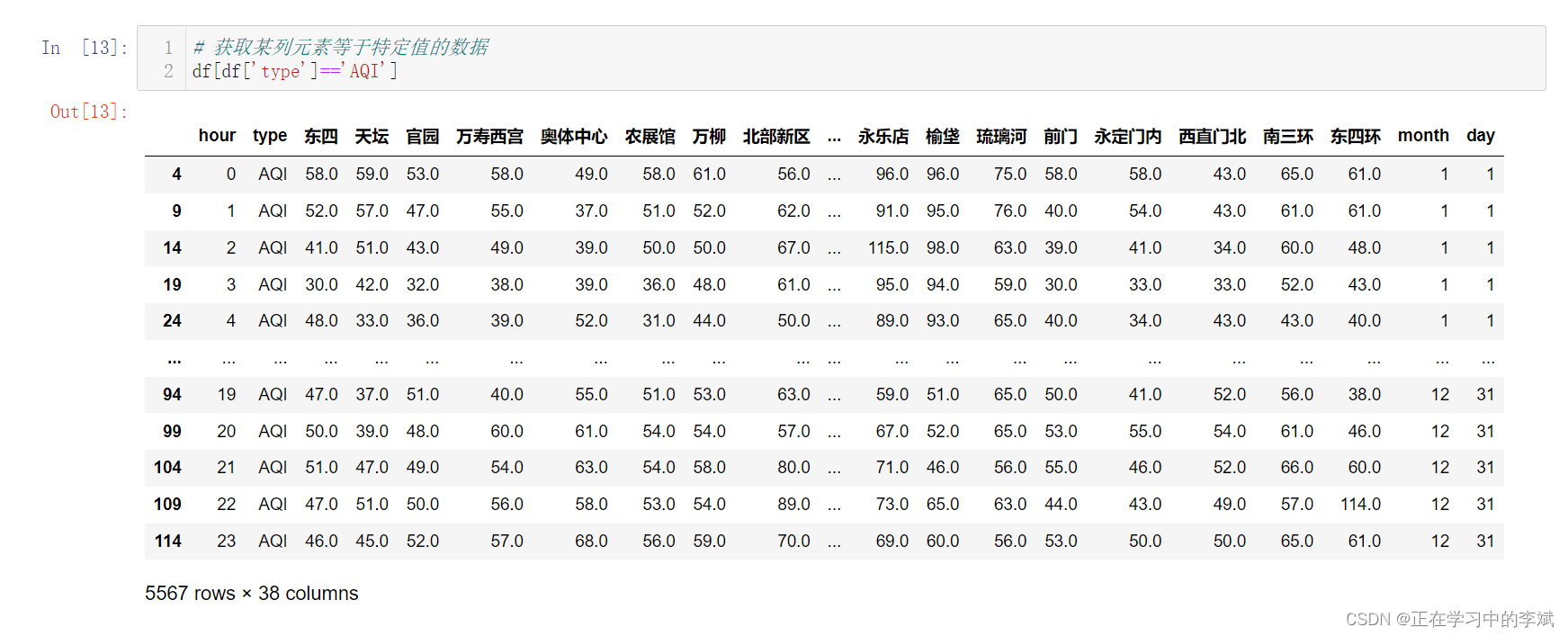# 二、聚合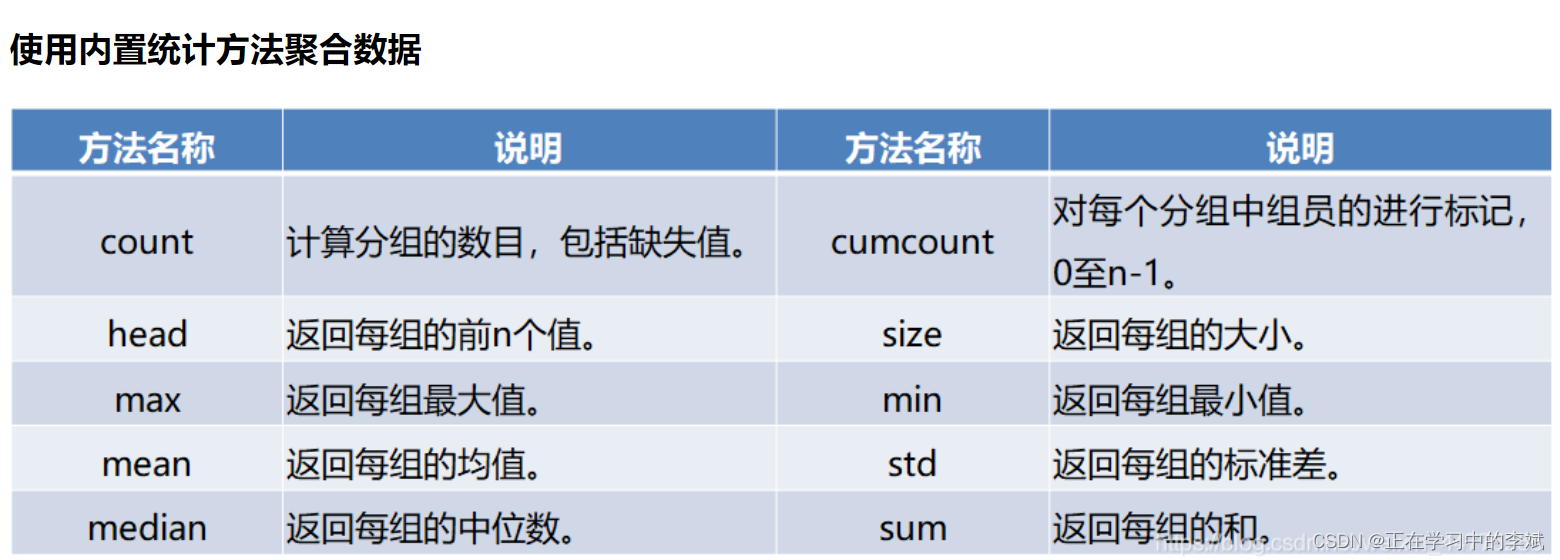##### 1. 使用内置函数聚合
``````# 对type列所有值等于AQI的数据进，行多重分组
group = df[df['type']=='AQI'].groupby(['hour', 'month'])
# 使用内置的方法，进行求平均值聚合，得到每个月每小事的平均值
group.mean()
``````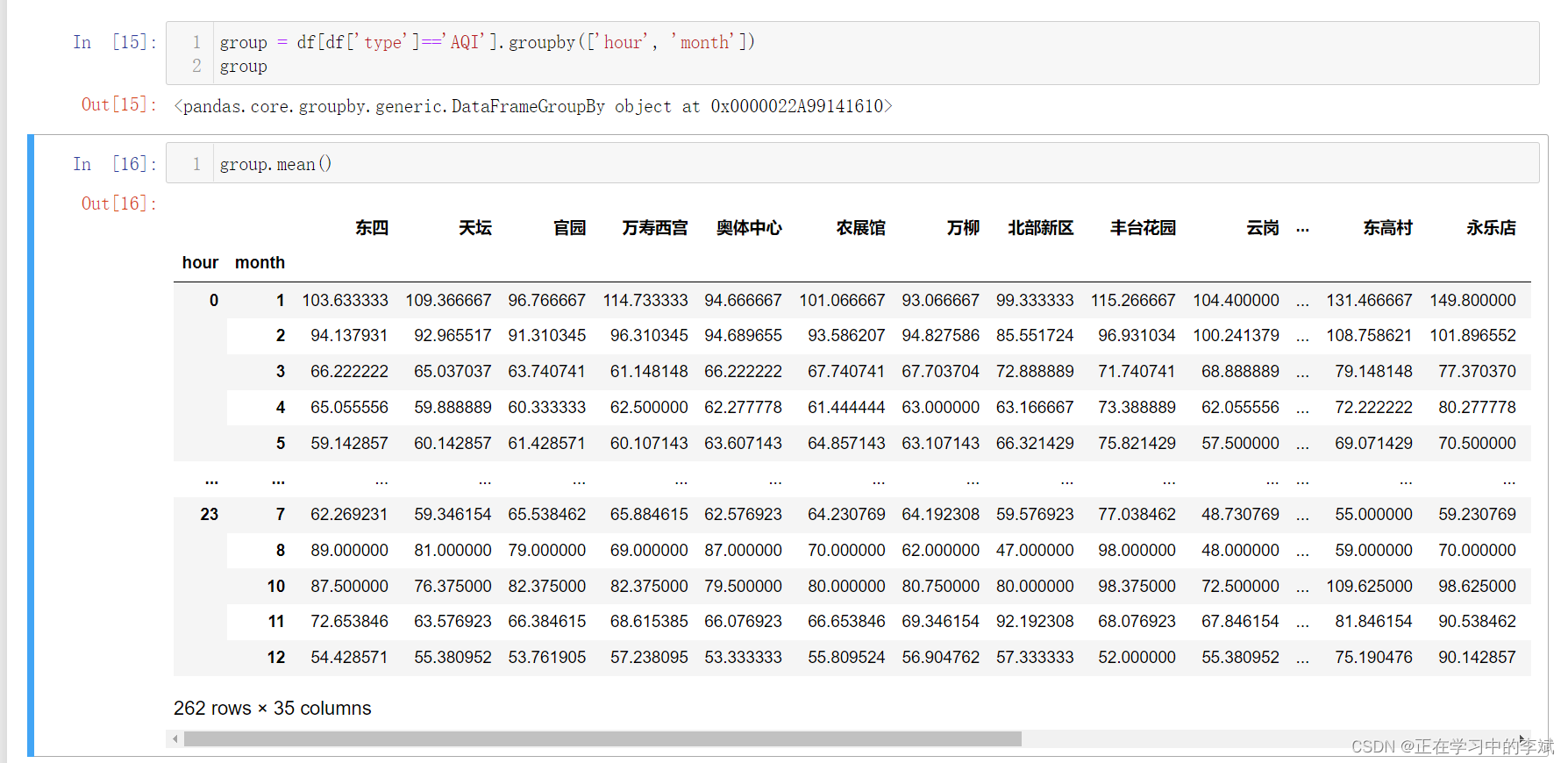##### 2. 利用agg进行简单的聚合
``````
# 对所有列数据应用相同函数的两个函数
group.agg([np.mean, np.std])

# 传入字典格式的数据
# 对不同列数据应用不同函数
group.agg({
'东四': [np.mean, np.std], '天坛': [np.min, np.max]})

``````

## 三、transform函数

``````import pandas as pd
import glob
import numpy as np

files = glob.glob('../../data/03表格数据处理Pandas/C3.7 数据的分组与聚合/*')
df = pd.concat([pd.read_csv(f) for f in files])
df = df.dropna(axis='columns', how='all').dropna(axis='index', how='any')
df['date'] = df.apply({
'date': lambda x: pd.to_datetime(x, format='%Y%m%d')})
df['month'] = df['date'].dt.month
df['day'] = df['date'].dt.day
df = df.drop(columns='date')
aqi = df[df['type']=='AQI']
# 改变数据形状
aqi.groupby(['month']).agg(np.mean)

# 不改变数据形状 应用内置函数
aqi.groupby(['month']).transform(np.mean)
# 不改变数据形状 应用匿名函数
aqi.groupby(['hour', 'month']).transform(lambda x: x - x.mean())
``````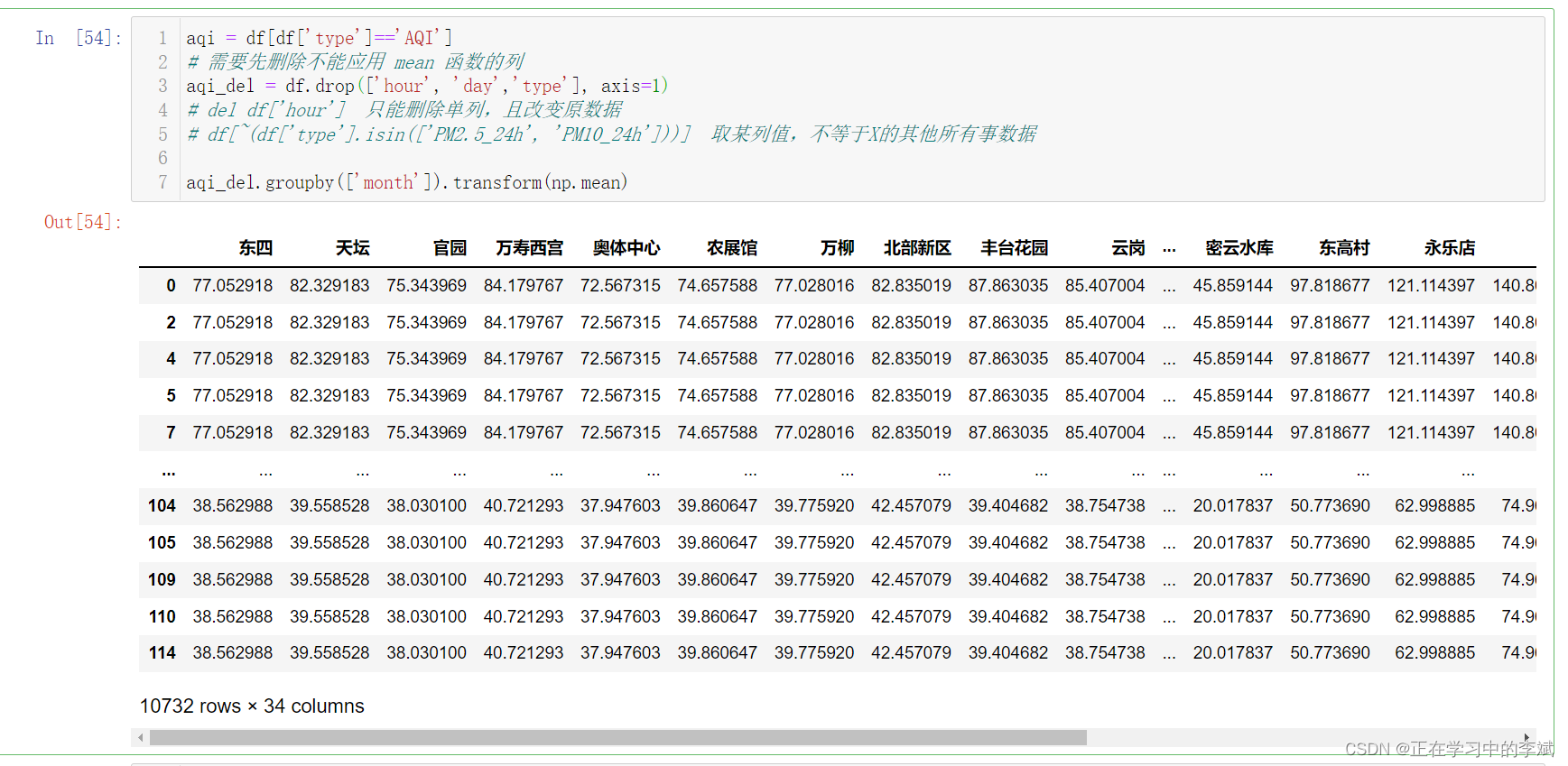### 一千行MySQL学习笔记（十一）_dingluan5041的博客-程序员秘密

--// 存储函数，自定义函数 ------------ 新建 CREATE FUNCTION function_name (参数列表) RETURNS 返回值类型 函数体 - 函数名，应该合法的标识符，并且不应该与已有的关键字冲突。 - 一个函数应该属于某个数据库，可以使用db_name.funciton_name的形式执行当前函数所属数据库，否则为...

### 分布式训练的GPU设置与分配（含源码可以直接测试）_gpu集群训练模型_ERROR_LESS的博客-程序员秘密

：输出日志信息，包含任务的布置情况 ：自动指定设备布置任务 ：设置可见设备，例如机器上有4个GPU，但设置只对一个GPU可见，则该进程无法访问其他设备 ：获取所有物理设备（整块） ：建立逻辑分区 ：获取所有逻辑设备（分块） ：设置内存自增长，需在程序开始的时候就被设置因此，本机有两块物理GPU先做一个默认gpu设置的实验，作为对照组。基础代码：容器内进行训练：默认情况下，此demo每步运行花费6ms。查看GPU占用情况：发现仅仅这一个进程就几乎占满GPU，对资源浪费十分严重。因此，进行

### 商用密码应用与安全性评估要点笔记（密码发展、密码算法）_搞搞搞高傲的博客-程序员秘密

Chrome基于RIng-LWE问题的抗量子密钥交换算法，微软公布其开发基于RIng-LWE问题的密钥交换算法的源代码。（引入噪声，当所需的乘法数量较少时，接近实用化）每次加密IV都必须重新生成，IV的引入使得对同一明文使用相同的密钥进行加密，得到不同的密文。1976年Diffle和Hellman发表《密码学的新方向》，首次证明公钥密码是可能的，提出了一种密钥协商的创造性方法（基于离散对数求解的困难问题）如PRINCE、SIMON、SPECK，分组长度32，48，64，80，96bit，补充空白。